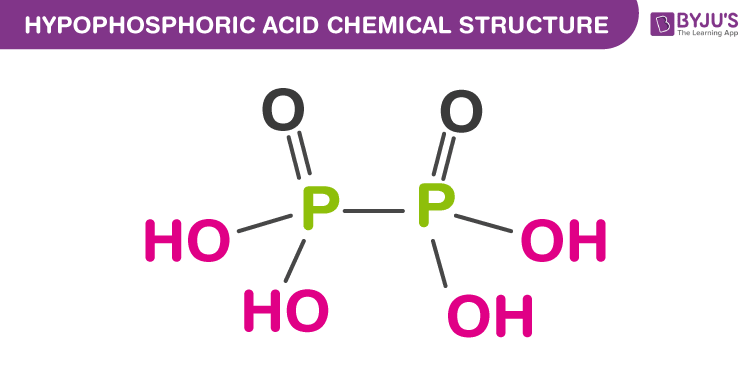# Hypophosphoric Acid Formula

Hypophosphoric acid is a mineral acid with phosphorus in an oxidation state of +4. It has a chemical formula H4P2O6. In the solid-state, it exists as a dihydrate, H4P2O6.2H2O. It can be manufactured by reacting red phosphorus with sodium chlorite at room temperature. In this short piece of article, let us discuss the hypophosphoric acid formula along with its chemical structure, properties and uses.

## Structure of Hypophosphoric Acid## Properties of Hypophosphoric Acid

 Properties of Hypophosphoric Acid Name Hypophosphoric Acid Other Names Diphosphoric Acid Appearance White Solid Chemical Formula H4P2O6 Melting Point 54 °C Molar Mass 161.98 g/mol Solubility in Water Soluble

## Preparation of Hypophosphoric Acid

• Hypophosphoric acid is prepared by hydrolysis and oxidation of red phosphorus by NaOCl, or white phosphorus by water and air.

2 P + 4 NaClO2 + 2 H2O →  H4P2O6 + 2 NaCl

• There are no P-H bonds and so this acid is not a reducing agent.
• It has four acidic hydrogens.
• It contains P-P bond.

## Uses of Hypophosphoric Acid

• Used as a bleaching agent
• Used as tetrabasic acid.
• Used as a wetting agent
• Used as a stimulant in pharmaceutical agents

### 1. What is the formula of Hypophosphoric acid?

The formula of hypophosphoric acid is H4P2O6. It contains four P-OH bonds, Two P=O bonds and one P-P bond.

### 2. What is the basicity of Hypophosphoric acid?

The structure of hypophosphoric H4P2O6 contains four P-OH bonds. Hence it has four acidic hydrogens and the basicity of hypophosphoric acid is 4.

### 3. What is the formula of hypophosphorous acid?

The formula of hypophosphorous acid is H3PO2. It contains one P-OH bond, one P=O bond and two P-H bonds.

### 4. What is the formula of Metaphosphoric acid?

The formula of Metaphosphoric acid is HPO3. It contains one P-OH bond, two P=O bonds.

### 5. What is the basicity of hypophosphorous acid?

The formula of hypophosphorous acid is H3PO2. It contains one P-OH the basicity of hypophosphorous acid is 1.